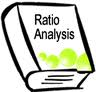Introduction:

The focus of financial analysis is on key figures in the financial statements and the significant relationship that exists between them. The analysis of financial statement is process of evaluating the relationship between component part of financial statements to obtain a better understanding of the firm’s positions and performance. In brief, financial analysis is the process of selection, relation and evaluation. What the ratios do is that they reveal the relationship in a more meaningful way so as to enable us to draw conclusion from them.

The rational of ratio analysis lies in the fact that it makes relative information comparable. A single figure by itself has no meaning but when express in terms of a related figure, its yields significant inferences.

Type of ratios

Liquidity ratios the importance of adequate liquidity in the sense of the ability of a firm to meet current/short term obligations when they become due for payment can hardly be overstressed. In fact, liquidity of is a prerequisite for the very servable of a firm.

We will separate 13 significant ratios in to four categories –

Profitability ratios

•  Profit margin
• Return on assets (investment)
• Return on equity
• Asset utilization ratios
• Receivable turn over
• Average collection period
• Inventory turnover
• Fixed asset turnover
• Total asset turnover
• liquidity ratios
• current ratios
• quick ratio debt
• utilization ratios
• Debt to total asset
•   Times interest earned
•   Fixed charge coverage

Current ratio

The current ratio is the ratio of total current assets to total current liabilities. It is calculated by dividing current asset by current liabilities.

Current ratio= current asset/ current liabilities

The current assets of a firm as already stated represent those assets which can be, in the ordinary course of business, converted into cash within a short period of time, normally not exceeding on year.

Current ratio of a firm measures its short term solvency that is its ability to meet short term obligations. It indicates the groups of current assets available for each group of current liability obligation. The higher the current ratio larger is the amount of groups available per group of current liability, the more is the form of ability to meet current obligation and the greater is the safety of funds of short term creditor.

Turnover Ratio

The liquidity ratios discussed so far relate to the liquidity of a firm as a whole. Another way of examining the liquidity is to determine how quickly certain current assets are converted into cash. The ratios to measure those are referred to as turnover ratios.

Inventory Turnover Ratios

It is computed by diverting the cost of good sols by the averaging inventory. Thus,

Inventory turnover ratio = cost of good sold / average inventory

The cost of goods sold means, sales – gross profit. The average inventory refers to the simple average of the opening and closing inventory. The ratio indicates how fast inventory sold.

Profitability ratio

Those ratios are on the promise that a firm should earn sufficient profit on each Taka of sale. If adequate profits are not earn on sale, there will be difficulty in meeting the operating expenses and no returns will be available to the owners. Those ratios consists of

• Profit margin.
• Expense ratios